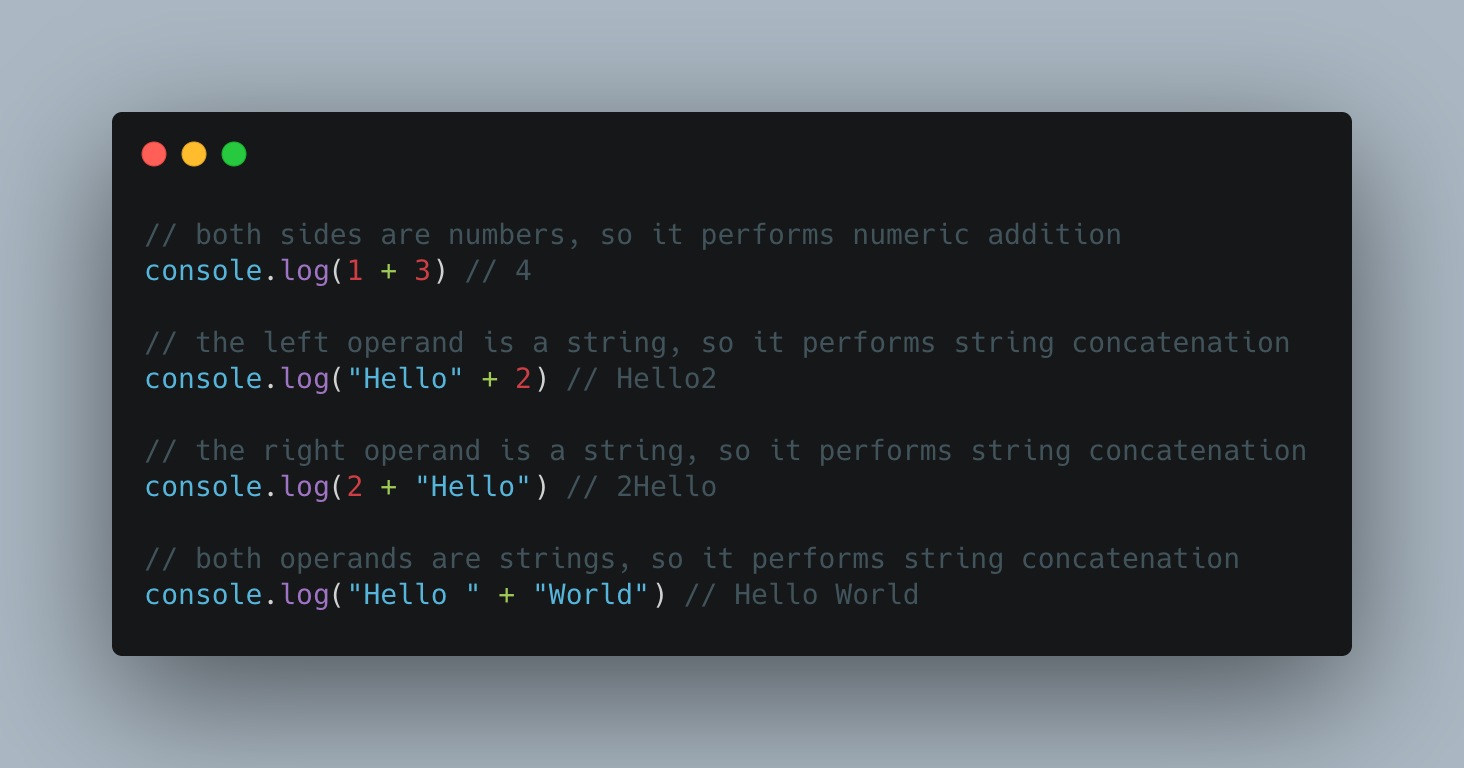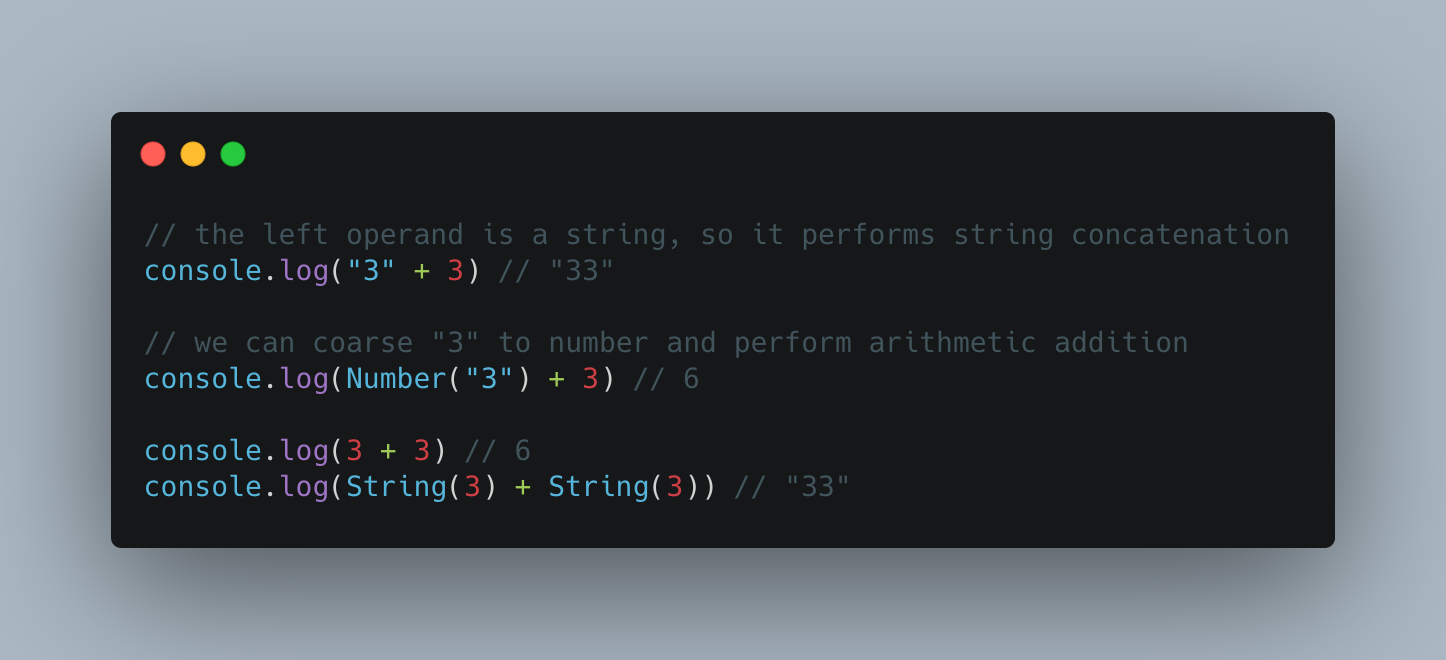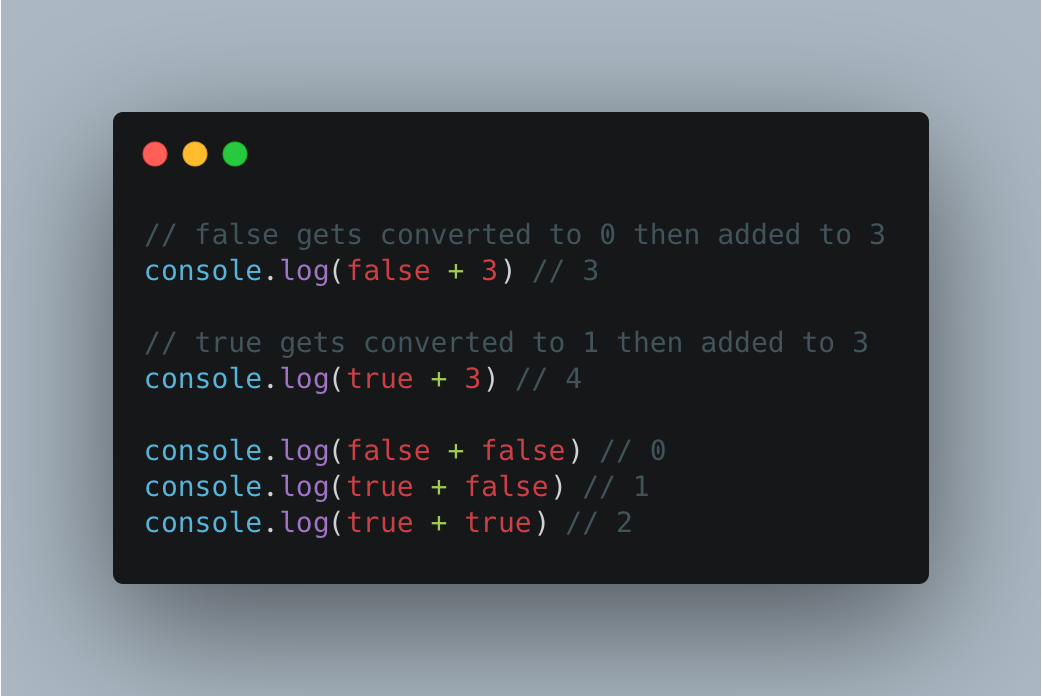# .css-4zleql{display:block;}Dev Notes by Tony# The Basics: JavaScript Addition Operator (+)

## Understanding the addition operator in JavaScript, with all it's nuances

Anthony Oyathelemhi
·Aug 30, 2021·

One of the uses of the plus (+) symbol in JavaScript is to perform addition of two values. It can either perform numeric addition, as you'd expect, but also string concatenation.

This is pretty standard in most programming languages. Let's take a brief look at both usages

``````console.log(15 + 5) // 20
``````

The code above logs 20 to the console. Straightforward

### String concatenation

``````console.log('Hello' + ' ' + 'World') // Hello World
``````

We get "Hello World" as the output, which is the result of appending "Hello", a blank space (" "), and "World". Again, straightforward

It wouldn't be JavaScript if things were this black and white now would it? That was rhetorical

## How JavaScript decides which operation to perform (the spec)

Since the addition operator can be used to do two things, the JS engine needs to somehow decide which one of the two to perform. This is well documented in the ECMAScript Spec but may not be easy to understand for most people. I have read it so you don't have to. In summary:

If any of the operands is a string, perform string concatenation, otherwise perform addition

Testing the ruleTo force numeric addition, you can use the build-in `Number()` constructor to coarse both operands to numbers. Similarly, you can use `String()` to force concatenationNOTE: apply caution when using Number(value) to convert values. If `value` isn't 'number-like', it will return `NaN`, which is something that deserves it's own blog post

## Boolean addition, sort of

Remember how I said it could only do numeric addition or string concatenation? That remains true. However, you can use any type whatsoever and the JS engine will try to convert the type to either number or string, before performing the operationWhy does the engine decide to convert the boolean values to numbers and not string? You might ask. To rephrase the rule

If both operands are not strings, convert the operands to numbers and perform a numeric addition

Unsurprisingly, `Number(false)` returns `0`, and `Number(true)` returns `1`

If you just started learning JavaScript and you've gotten this far, first of all, Good job! You can stop here because the next section might confuse you even more

Up on till now, we've only looked at adding primitive values, three of seven in JavaScript. Since JavaScript is a loosely typed language, there's nothing stopping us from doing this

``````[] + {}
7 + []
{} + ""
``````

The JavaScript engine has to first convert all operands to Primitive types, then decides whether to perform string concatenation or numeric addition. Let us expand on the summary I provided at the beginning of this blog post to understand what's going on.

A simplified version of what the runtime does under the hood

``````function add(leftValue, rightValue) {
var leftPrimitive = toPrimitive(leftValue)
var rightPrimitive = toPrimitive(rightValue)

if (typeof leftPrimitive === 'string' || typeof rightPrimitive === 'string') {
return String(leftPrimitive) + String(rightPrimitive)
} else {
return Number(leftPrimitive) + Number(rightPrimitive)
}
}
``````

And here we define the `toPrimitive` function

``````function toPrimitive(value) {
if (typeof value === 'object') {
let primitiveOptionOne = value["valueOf"]();
let primitiveOptionTwo = value["toString"]();

if (typeof primitiveOptionOne !== 'object') {
return primitiveOptionOne
} else if (primitiveOptionTwo !== 'object') {
return primitiveOptionTwo
}

// otherwise we'll end up in an endless loop
throw new TypeError('Cannot convert object to primitive value')
} else {
return value
}
}
``````

In simple English

1. Convert both Operands to their primitive types by calling the built-in ToPrimitive abstract operator
2. If any of the primitives in the previous step is a string, do string concatenation, otherwise continue
3. Convert both Operands to numbers and perform numeric addition

Based on what we've learned so far, we can make the following deductions

``````3 + 3 ==> Number(3) + Number(3) ==> 6

"Hello" + 3 ==> String("Hello") + String(3) ==> "Hello3"

7 + [] ==> String(7) + String([]) ==> "7"

[] + {} ==> String([]) + String({}) ==> "[object Object]"

{} + "4" ==> String({}) + String("4") ==> "[object Object]4"

false + 2 ==> Number(false) + Number(2) ==> 2

true + 3 ==> Number(true) + Number(3) ==> 4
``````

To test that the JS engine does in fact call `toValue()` within the `toPrimitive()` call, open a new browser terminal (or head to playcode.io/new) and run the following code

``````// DO NOT TRY THIS AT HOME
Object.prototype.valueOf = () => 5

console.log({} + 4) // 9
``````

Unsurprisingly, we get 9, yay!

Got questions, suggestions, head over to the comment section let's have a chat

tc39.es/ecma262/multipage/ecmascript-langua..

developer.mozilla.org/en-US/docs/Web/JavaSc..